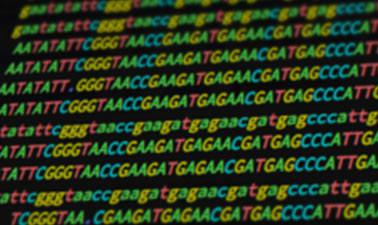## Sunday, March 22, 2020

### Statistical Inference and Modeling for High-throughput ExperimentsIn this course you&rsquo;ll learn various statistics topics including multiple testing problem, error rates, error rate controlling procedures, false discovery rates, q-values and exploratory data analysis. We then introduce statistical modeling and how it is applied to high-throughput data. In particular, we will discuss parametric distributions, including binomial, exponential, and gamma, and describe maximum likelihood estimation. We provide several examples of how these concepts are applied in next generation sequencing and microarray data. Finally, we will discuss hierarchical models and empirical bayes along with some examples of how these are used in practice. We provide R programming examples in a way that will help make the connection between concepts and implementation. Given the diversity in educational background of our students we have divided the series into seven parts. You can take the entire series or individual courses that interest you. If you are a statistician you should consider skipping the first two or three courses, similarly, if you are biologists you should consider skipping some of the introductory biology lectures. Note that the statistics and programming aspects of the class ramp up in difficulty relatively quickly across the first three courses. By the third course will be teaching advanced statistical concepts such as hierarchical models and by the fourth advanced software engineering skills, such as parallel computing and reproducible research concepts. These courses make up 2 XSeries and are self-paced: PH525.1x: Statistics and R for the Life Sciences PH525.2x: Introduction to Linear Models and Matrix Algebra PH525.3x: Statistical Inference and Modeling for High-throughput Experiments PH525.4x: High-Dimensional Data Analysis PH525.5x: Introduction to Bioconductor: annotation and analysis of genomes and genomic assays PH525.6x: High-performance computing for reproducible genomics PH525.7x: Case studies in functional genomics This class was supported in part by NIH grant R25GM114818. &nbsp;